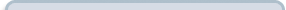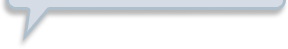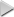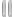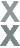# Fan Appreciation Night, Part 1

###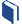Resources for this lesson: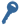Key Terms:

Experimental probability

> Glossary> Calculator Resources> Teacher Resources: Instructional NotesLet’s go back to Khalid’s situation: What is the probability that he would answer all 8 history questions correctly? There are two ways to investigate this probability.  One way is to create a simulation to calculate the experimental probability of answering each of the questions correctly.

## Create and Analyze

There are a number of ways to design and run a simulation.  First, select a model that can represent answering 80% of the questions correctly, such as:

A Spinner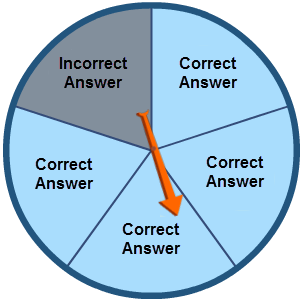• Includes 5 equal sections, 4 of which represent correct answers.
• Spin 8 times with each spin representing answering one of the 8 history questions asked during the It’s Academic Tournament.
• The results of the 8 spins would make up one trial for this simulation.
• Run at least 10 trials.

Random Integer Generator

• Use random digits 1 - 5.
• 1 - 4 represent correct answers.
• Generate 8 digits for each trial. Each digit represents answering one of the 8 History questions in a typical It's Academic Tournament.
• Run at least 10 trials.